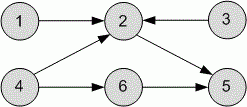Competitions

The smallest Topological Sort

The directed unweighted graph is given. Find the lexicographically smallest topological ordering of its vertices.

Input

The first line contains the number of vertices n (1n105) and the number of edges m (1m105) in a graph. Each of the next m lines describes the edge of the graph - two numbers, the initial and final vertex.

Output

Print the lexicographically smallest topological ordering of graph's vertices. If its impossible to sort graph topologically, print -1.Time limit 1 second
Memory limit 128 MiB
Input example #1
6 6
1 2
3 2
4 2
2 5
6 5
4 6
Output example #1
1 3 4 2 6 5
Author Michael Medvediev